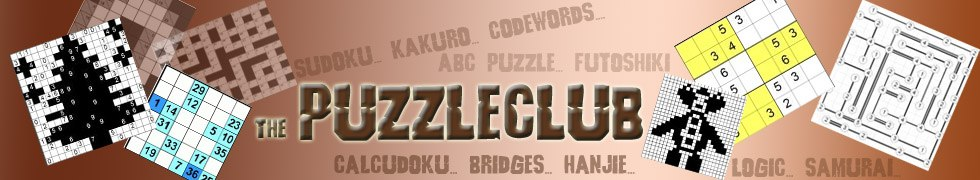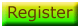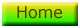## Kakuro Instructions

A kakuro puzzle will look like the one below, but of course bigger: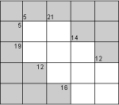Each kakuro puzzle is composed of a number of rows and columns, some cells are empty and light coloured, others some have numbers in them. At first look therefore it looks like a crossword.

Every sequence of cells in either a row or a column is called a 'run'. At the left of each horizontal run, and at the top of each vertical run, you will see a number.

In the image above, you can see, for instance, a '5' in the second cell on the top row. This means simply that the sum of the two cells underneath it is 5. Next to the '5' is '21' - this means, therefore, that the three cells underneath it sum to 21. And so on, throughout the puzzle.

Using this information alone, you can solve a kakuro puzzle. The key constraint is that you can only use a number once in each run. Therefore if you need to make '6' from two numbers, you cannot use '3' twice, but must rather use 1 and 5 or 4 and 2. You may only use the numbers 1 to 9.

# Kakuro Hints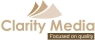©   |     |